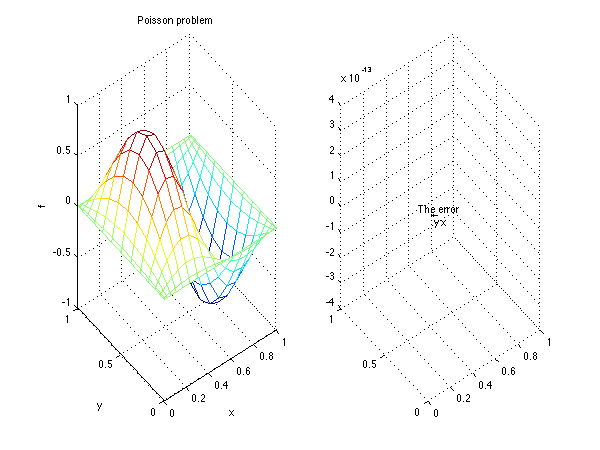# Poisson problem in 2D

(We do this in 3D in poisson3D.m)

Here we solve a Poisson problem in 2D to give an example of the differentiatino matrices and the boundary conditions in 2D. This is the same example as done in the Poisson test case of gerris.

clear all; clf

%%%% parameters and flags
Nx=20; % gridpoints in x
Ny=15; % gridpoints in x
Lx=1; % domain size in x
Ly=1; % domain size in y

% 1D and 2D differentiation matrices
[d.x,d.xx,d.wx,x]=dif1D('cheb',0,Lx,Nx,3);
[d.y,d.yy,d.wy,y]=dif1D('cheb',0,Ly,Ny,3);
[D,l,X,Y,Z,I,NN]=dif2D(d,x,y);

% System matrix
A=D.xx+D.yy;


# The exact solution

We do a Poisson problem with homogeneous boundary conditions at all boundaries (the value of f is zero), but with a forcing term b \displaystyle \Delta f=b and the forcing is \displaystyle b=-\pi^2(k^2+l^2)sin(\pi k x) sin( pi l y) and the exact solutino is \displaystyle f=sin(\pi k x)sin(\pi l y) This solution is easy to check by doing a Fourier transform. We suppose we have an harmonic solution \displaystyle f(x,y)=\hat{f}\exp(i\pi kx+i\pi ly)+\text{Complex conjugate} which is fine as well as the boundary conditions that we impose fit with this (which is the case here). We have \displaystyle f_x=-i\pi k \hat{f} \exp(...)+C.C. and \displaystyle f_x=-i\pi l \hat{f} \exp(...)+C.C, thus \displaystyle (-\pi^2k^2-\pi^2l^2)\hat{f}\exp(...)=-\pi^2(k^2+l^2)\exp(...) thus \hat{f}=1.

% Forcing
k=2; ell=1;
b=-pi^2*(k^2+ell^2)*sin(pi*k*X).*sin(pi*ell*Y);
b=b(:);


# Imposing the boundary conditions

To impose the boundary conditions, we replace the lines of A with the lines of the identity matrix corresponding to the elements on the boundaries, and we replace the boundary elements of the forcing term with zeros. Doing this we effectively impose that the values of f at the boundary cells must be zero.

To impose these boundary conditions we use locations vectors which contain the indices of the cells correponding to the different boundaries of the mesh. Please see pedagogy#location-vectors-and-matrices to learn about locations. These are stored in the different fields of the structure l. To see how l is built, please see dif2D.m#location-vectors.

% boundary conditions
II=eye(Nx*Ny); ZZ=zeros(Nx*Ny,Nx*Ny);
loc=[l.top; l.bot; l.left; l.right];
A(loc,:)=II(loc,:);
b(loc)=0;

% solving the linear system
f=A\b;

% plotting the result
subplot(1,2,1);
mesh(X,Y,reshape(f,Ny,Nx));
xlabel('x'); ylabel('y'); zlabel('f')
title('Poisson problem');

subplot(1,2,2);
solexact=sin(pi*k*X).*sin(pi*ell*Y);
mesh(X,Y,reshape(f,Ny,Nx)-solexact);
xlabel('x'); ylabel('y'); zlabel('f')
title('The error');Poisson solution and error

# Exercices/contributions

• Please do a convergence study with the grid
• Please find an other validation case poisson_2D_othervalidation.m
• Please change the boundary conditions (for instance non-homogeneous Dirichlet)